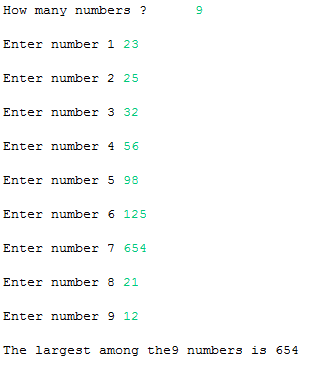## Thursday, 8 January 2015

### Java Program to find the Largest Element in an Array

This is a Java Program which finds the largest element in an array.

Here initially first element in array and  its next element are compared array and array , if array is greater then the value is stored in array and now the second element is increased 2 .3 4 .. until the last element is reached.

PROGRAM :
```package codingcorner.in;

import java.util.Scanner;

public class LargestElementArray {
public static void main(String[] args) {
int i, n;
System.out.print("How many numbers ?\t");
Scanner scan = new Scanner(System.in);
n = scan.nextInt();
int array[] = new int[n];
for (i = 0; i < n; i++) {
System.out.print("\nEnter number " + (i + 1));
array[i] = scan.nextInt();
}
scan.close();
for (i = 0; i < n; i++) {
if (array < array[i])
array = array[i];
}
System.out.print("\nThe largest among the" + n + " numbers is "
+ array);
}
}```

OUTPUT :Java - Largest Element in an Array

Related Programs:
Sum Of Elements in an array ( Cpp )
Sum Of Elements in an array ( Java)
Largest Element in an array( C )
Largest Element in an array ( Cpp )
Sum Of Elements in an array (C)